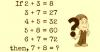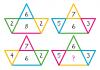BRAIN TEASERSBrain Teasers User Profile

# Mondher Romdhani

rank
317
points
9
See full ranking list
short ranking list
 315 JAYANTA KUMAR DAS ADHIKARI 9 316 Robert Garrigos 9 317 Mondher Romdhani 9 318 Tarek Ibrahim 9 319 Israel Omar Leon Magne 9
 Replace the question mark with a number MATH PUZZLE: Can you replace the question mark with a number?Solve Math Puzzle If 2 + 3 = 8, 3 + 7 = 27, 4 + 5 = 32, 5 + 8 = 60, 6 + 7 = 72, then, 7 + 8 = ?Replace the question mark with a number MATH PUZZLE: Can you replace the question mark with a number?